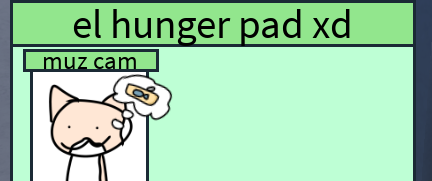# Event Not Triggering When An IntValue Is At A Specific Point

1. What do you want to achieve? Me and my friends are making a game based on the game “raise a floppa”. We are making a GUI that tells you when your muz (the thing you’re raising) is at a specific hunger.

2. What is the issue? My friend made this script (script is at the end). But for some reason it wont work

Video of the problem

What its supposed to show1. What solutions have you tried so far? I modified the script to make it so if its less or equal to that value.
``````local value = game.Workspace.muz.Head.Hunger
local image = script.Parent.muz
local yum = script.Parent.yum
--// -----------------------------------------

-- the mmm fujnny

-- el value manager

if value.Value <= 99 then

image.Image = 9641894200
yum.ImageTransparency = 1
end

if value.Value <= 70 then
yum.ImageTransparency = 0
end

if value.Value <= 60 then
image.Image = 9641893835
end

if value.Value <= 40 then
image.Image = 9641893700
end

if value.Value <= 25 then
image.Image = 9641893452
end
-- P.S: the id change doesnt work either
``````

Any fix for this?

The problem is that you’re only checking once, you should either put this in a loop (not recommended), or connect it to a property changed event

The script below probably solves it. It basically just connects your function to the event that gets triggered when the hunger value changes.

``````local value = game.Workspace.muz.Head.Hunger
local image = script.Parent.muz
local yum = script.Parent.yum
--// -----------------------------------------

-- the mmm fujnny

-- el value manager

function check()
if value.Value <= 99 then
image.Image = 9641894200
yum.ImageTransparency = 1
end

if value.Value <= 70 then
yum.ImageTransparency = 0
end

if value.Value <= 60 then
image.Image = 9641893835
end

if value.Value <= 40 then
image.Image = 9641893700
end

if value.Value <= 25 then
image.Image = 9641893452
end
end

value.Changed:Connect(check)
``````

Oh yeah. I don’t think placing a number instead of a string will work.

Don’t let your friend be the next YandereDev and make him use tables.

``````local value = workspace.muz.Head.Hunger
local image = script.Parent.muz
local yum = script.Parent.yum
--// -----------------------------------------

-- the mmm fujnny

-- el value manager

local hungerStates = {
 = "rbxassetid://9641894200",
 = "rbxassetid://9641893835",
 = "rbxassetid://9641893700",
 = "rbxassetid://9641893452",
}

function check()
local minimums = {}

for decrease, id in pairs(hungerStates) do
if value.Value <= decrease then
table.insert(minimums, decrease)
end
end

if #minimums > 0 then
local min = math.min(table.unpack(minimums))

yum.Image = hungerStates[min]
end

if value.Value < 100 then
yum.ImageTransparency = 1
end

if value.Value <= 70 then
yum.ImageTransparency = 0
end
end

value.Changed:Connect(check)
``````

Thanks for solving this. I didn’t know the last line was a thing until now! (i had to modify the script a little because yum is the thought bubble in the image below the video but thanks for solution)

I tried this code but unfortunately the muz image disappears.